# Lattice Method of Multiplication

An error occurred trying to load this video.

Try refreshing the page, or contact customer support.

Coming up next: What Are Different Ways to Multiply?

### You're on a roll. Keep up the good work!

Replay
Your next lesson will play in 10 seconds
• 0:04 What Is the Lattice Method?
• 0:43 Using Multiplication Tables
• 1:13 Lattice Method
• 2:39 Practice
• 3:14 Lesson Summary
Save Save

Want to watch this again later?

Timeline
Autoplay
Autoplay
Speed Speed

#### Recommended Lessons and Courses for You

Lesson Transcript
Instructor: Danielle Wilson

Danielle is a certified elementary, middle school math, and special education teacher. She has a master's degree in elementary education and special education.

Long multiplication can be... long! The good news is that there are different ways you can multiply numbers with two or more digits without the long multiplication model. In this lesson, we will discuss the lattice method and how it can be useful when multiplying multi-digit numbers.

## What Is the Lattice Method?

The lattice method is an alternative way to do multiplication. The lattice method got its name from using a type of grid with a diagonal criss-cross pattern called a lattice. Can you think of an example of a lattice in the real world? Maybe Grandma's yummy lattice apple pie?

You might have heard that the lattice method is a new way to multiply, but the truth is the lattice method has been around since the Middle Ages, or even before (and that's way before your parents were born!).

The lattice method helps organize the process of long multiplication into smaller steps. It can be complicated at first but with practice you might find that multiplying large numbers can be fun!

## Using Multiplication Table

Let's explore the lattice method by first multiplying with a multiplication table. Start with the following problem: 35 x 12.

If you wrote 35 x 12 in expanded form, we would get (30 + 5) x (10 + 2). You can use a multiplication table to get your answer.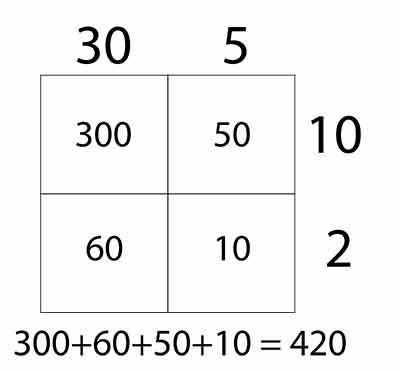The multiplication table is useful when multiplying smaller numbers, but not with larger numbers. The lattice method can help with this by dropping extra zeroes and splitting each box into a tens and ones place.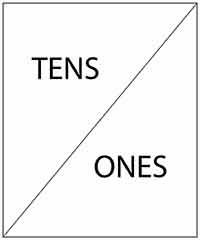## Lattice Method

Let's multiply 35 x 12 again using the lattice method. Now you will multiply each corresponding row with each column and write your answer in the boxes.

Draw rows and columns based on the number of digits in each factor. Draw diagonal lines through each of the boxes. Write the first factor along the top columns and the second factor down the side of the rows.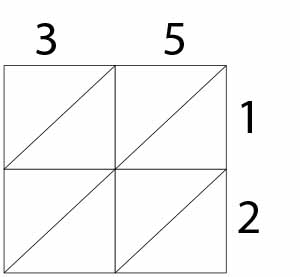Begin by multiplying each row digit by each column digit. Start by multiplying 5 x 1. That equals 5, so put a 5 in the ones section and a 0 in the tens section, since there is no tens value.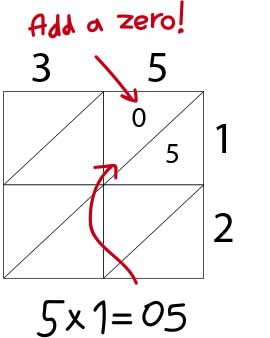Repeat the first step until you complete the lattice. Every row number across is multiplied by every column number down.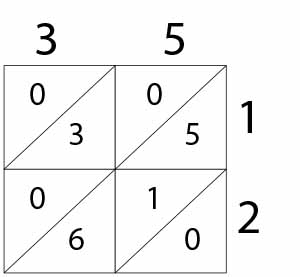To unlock this lesson you must be a Study.com Member.

### Register to view this lesson

Are you a student or a teacher?

#### See for yourself why 30 million people use Study.com

##### Become a Study.com member and start learning now.
Back
What teachers are saying about Study.com

### Earning College Credit

Did you know… We have over 200 college courses that prepare you to earn credit by exam that is accepted by over 1,500 colleges and universities. You can test out of the first two years of college and save thousands off your degree. Anyone can earn credit-by-exam regardless of age or education level.